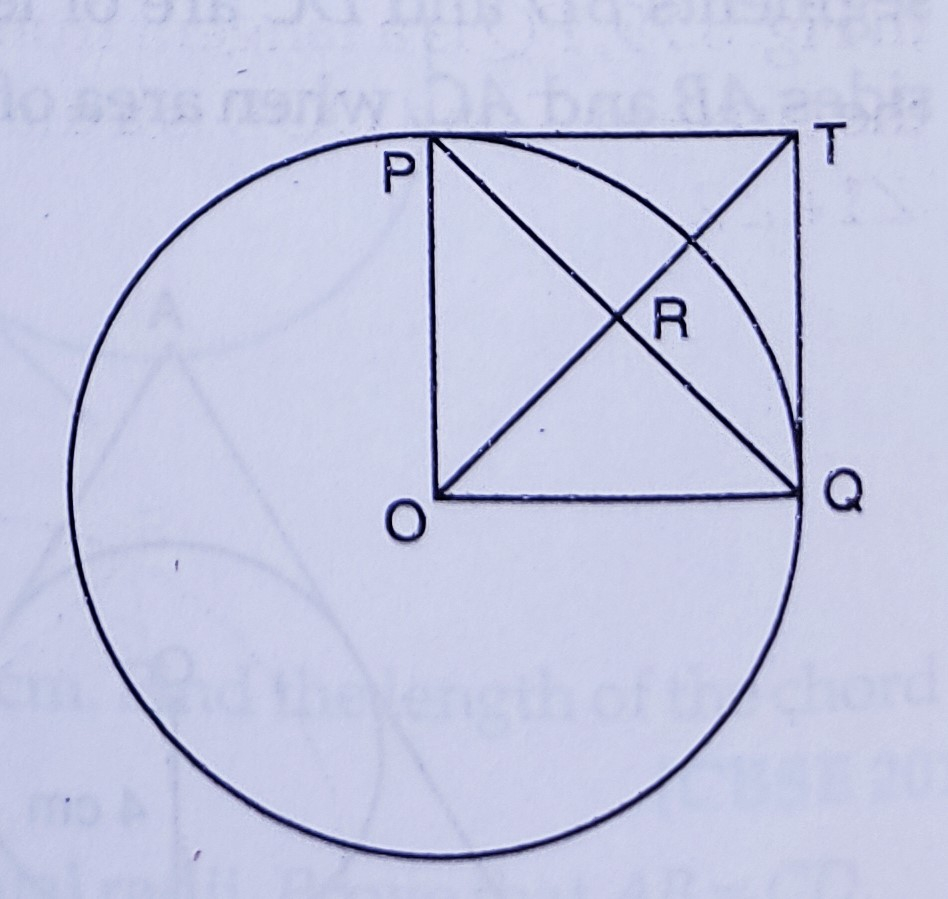"
">

# In the figure $P O \perp Q 0$. The tangents to the circle at $P$ and $Q$ intersect at a point $T$. Prove that $P Q$ and $O T$ are right bisectors of each other."

Given:

In the figure $P O \perp Q 0$. The tangents to the circle at $P$ and $Q$ intersect at a point $T$.

To do:
We have to prove that $P Q$ and $O T$ are right bisectors of each other.

Solution:

$PT$ and $QT$ are tangents to the circle.

$PT = QT$

$PO\ \perp\ QO$

$OP$ and $OQ$ are radii of the circle and $\angle POQ = 90^o$

$OQTP$ is a square where $PQ$ and $OT$ are diagonals.

Diagonals of a square bisect each other at right angles.

$PQ$ and $OT$ bisect each other at right angles.

Therefore, $PQ$ and $QT$ are right bisectors of each other.

Hence proved.

Updated on: 10-Oct-2022

38 Views